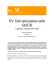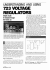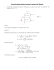# Thevenin`s and Norton`s Equivalent Circuit

## Transcription

Thevenin`s and Norton`s Equivalent Circuit
```Thevenin’s and Norton’s Equivalent Circuit Tutorial. (by Kim, Eung)
Thevenin's Theorem states that we can replace entire network by an equivalent circuit that
contains only an independent voltage source in series with an impedance (resistor) such that
the current-voltage relationship at the load is unchanged.
Norton's Thereom is identical to Thevenin's Theorem except that the equivalent circuit is an
independent current source in parallel with an impedance (resistor). Therefore, the Norton
equivalent circuit is a source transformation of the Thevenin equivalent circuit.
Original
Circuit
Norton‘s
Equivalent
Circuit
Thevenin’s
Equivanlent
Circuit
They are
Interchangeable
1
How to find Thevenin's Equivalent Circuit?
If the circuit contains
You should do
1) Connect an open circuit between a and b.
2) Find the voltage across the open circuit which is Voc.
Voc = Vth.
Resistors
and
independent sources
3) Deactivate the independent sources.
Voltage source Î open circuit
Current source Î short circuit
4) Find Rth by circuit resistance reduction
1) Connect an open circuit between a and b.
2) Find the voltage across the open circuit which is Voc.
Voc = Vth.
Resistors
and
dependent sources
or
independent shorces
If there are both dependent and independent sources.
3) Connect a short circuit between a and b.
4) Determine the current between a and b.
5) Rth = Voc / Iab
If there are only dependent sources.
3) Connect 1 Ampere current source flowing from
terminal b to a. It = 1 [A]
4) Then Rth = Voc / It = Voc / 1
Note: When there are only dependent sources, the equivalent network is merely RTh, that is, no
current or voltage sources.
2
How to find Norton's Equivalent Circuit?
If the circuit contains
You should do
Resistors
and
independent sources
- Deactivate the independent sources.
Voltage source Î open circuit
Current source Î short circuit
- Find Rt by circuit resistance reduction
- Connect an short circuit between a and b.
- Find the current across the short circuit which is Isc.
1) Connect a short circuit between a and b.
2) Find the current across the short circuit which is Isc.
Isc = In.
Resistors
and
dependent sources
or
Independent sources
If there are both dependent and independent sources.
3) Connect a open circuit between a and b.
4) Determine the voltage between a and b. Voc = Vab
5) Rn = Voc / Isc
If there are only dependent sources.
3) Connect 1 Ampere current source flowing from
terminal b to a. It = 1 [A]
4) Then Rn = Voc / It = Voc / 1
Note: When there are only dependent sources, the equivalent network is merely RTh, that is, no
current or voltage sources.
References
1. Introduction to Electric Circuits 5th Edition. Richard C. D and James A. S. 2001. John Wiley & Sons, Inc.
3
```

### Why try Circuit?### Alexander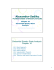### Low Cost Pure Sine Wave Solar Inverter Circuit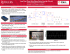### Appendix A Thevenin`s Theorem - Department of Physics | Oregon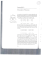### TB-CDP02-TX/RX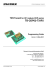### Thevenin equivalent circuits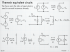### The Beat Goes On - Room 217 Foundation### Experiment 17: Kirchhoff`s Laws for Circuits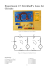### Chapter 20: AC Network Theorems### The Hypnotizer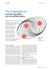### Router High-Flex Green Efficiency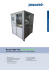### TDA2040 - Micropik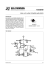### • Kirchhoff`s Laws and Basic Circuit • Energy and Power • Resistors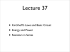### Exercises Unit 5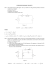### Circuit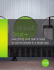### TDA2050 - 32W Hi-Fi Audio Power Amplifier Datasheet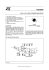### July 2016 - Masters Electrical Services, LTD### electrical products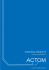### Parallel Operation of Turbo-Compressors in a Common System### PV Cell simulation with QUCS